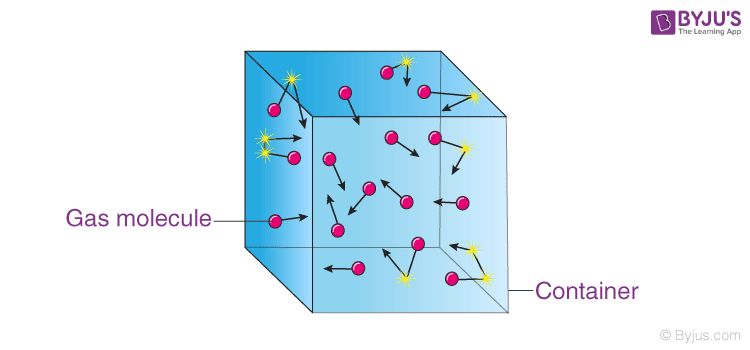# Kinetic Theory Of Gases: Assumptions

The behaviour of gases is studied by considering the small scale action of individual molecules or by considering the large scale action of the gas as a whole. The large scale action of the gas can be easily studied and measured, however, to study the action of the gas molecules a theoretical model needs to be used. This theoretical model is known as the kinetic theory of gases. In this article let us discuss the kinetic theory of gases and the assumptions considered for the theory.

## What is the Kinetic Theory of Gases?

In the 19th century, scientists James Clark Maxwell, Rudolph, and Clausius developed the kinetic theory of gases in order to explain the behaviour of gases. The theory explains gas as a collection of tiny, hard spheres that interact with each other and with the surface of the wall. The spheres represent the gas molecules, and they behave according to the laws of motion developed by Newton in the 17th century.  It describes how molecules influence gas characteristics such as temperature and pressure. It also explains why gases follow Boyle’s law.

We have learned that the pressure (P), volume (V), and temperature (T) of gases at low temperature follow the equation:

$$PV$$ = $$nRT$$

Where, n = number of moles in the gas and R = gas constant having value $$8.314 JK^{-1}mol^{-1}$$

Now, any gas which follows this equation is called an ideal gas. Hence, the equation is known as the ideal gas equation. But there are certain assumptions that we consider for describing ideal gas behaviour.## Assumptions of Kinetic Theory of Gases

Following are the kinetic theory of gases assumptions:

• All gases are made up of molecules that are constantly and persistently moving in random directions.
• The separation between the molecules is much greater than the size of molecules.
• When a gas sample is kept in a container, the molecules of the sample do not exert any force on the walls of the container during the collision.
• The time interval of a collision between two molecules, and between a molecule and the wall is considered to be very small.
• All the collisions between molecules and even between molecules and walls are considered to be elastic.
• All the molecules in a certain gas sample obey the laws of motion.
• If a gas sample is left for a sufficient time, it eventually comes to a steady state. The density of molecules and the distribution of molecules are independent of position, distance, and time.

### Postulates of Kinetic Theory of Gases

Following are the kinetic theory of gases postulates:

• The space-volume to molecules ratio is negligible.
• There is no force of attraction between the molecules at normal temperature and pressure. The force of attraction between the molecules builds when the temperature decreases and the pressure increases.
• There is a large space between the molecules resulting in continuous motion.
• The free movement of molecules results in a collision which is perfectly elastic.
• The molecules have kinetic energy due to random movement. But the average kinetic energy of these molecules differs with temperature.
• Molecules exert pressure on the walls of the container.

### What are the three main components of the kinetic theory of gas?

Following are the three main components of the kinetic theory of gas:

• When molecules collide with each other, no energy is gained or lost.
• The space occupied by the molecules of gas in a container is very negligible.
• These molecules always have linear motion.

Related Articles:

## Frequently Asked Questions – FAQs

### What is the kinetic theory of gas?

Kinetic theory explains the behaviour of gases based on the idea that the gas consists of rapidly moving atoms or molecules. This is possible as the interatomic forces between the molecules are neglected in gas.

### Who developed the kinetic theory of gases?

In the 19th century, scientists James Clark Maxwell, Rudolph, and Clausius developed the kinetic theory of gases in order to explain the behaviour of gases.

### Why is the kinetic theory of gases important?

The kinetic theory of gases explains the macroscopic properties of gases, such as volume, pressure, and temperature.

### What are the three main components of the kinetic theory of gases?

The three main components of the kinetic theory of gases are: 1) When molecules collide with each other, no energy is gained or lost. 2) The space occupied by the molecules of gas in a container is very negligible. 3) These molecules always have linear motion.

### What are the assumptions made in the kinetic theory of gases?

1) All gases are made up of molecules that are constantly and persistently moving in random directions. 2) All the collisions between molecules and even between molecules and walls are considered to be elastic. 3) All the molecules in a certain gas sample obey Newton’s laws of motion.

Test your knowledge on Kinetic theory of gases assumptions